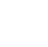# Java Operators

An operator is a symbol that tells the computer to perform certain mathematical or logical manipulations. Operators are used in programs to manipulate data and variables.

## Types of Operators

i) Arithmetic Operators

Java arithmetic operators are used to perform addition, subtraction, multiplication, and division. They act as basic mathematical operations.

Arithmetic Operators

Example:

class Example {
public static void main(String args[]) {
int num1 = 4;
int num2 = 2;
System.out.println(num1 + num2); //6
System.out.println(num1 - num2); //2
System.out.println(num1 * num2); //8
System.out.println(num1 / num2); //2
System.out.println(num1 % num2); //0

}
}

ii) Unary Operator

The Java unary operators require only one operand. This operand comes either before or after the operator.

The Java unary operators require only one operand. Unary operators are used to performing various operations i.e.:

1.Post-increment:

A post-increment operator is used to increment the value of the variable after executing the expression completely. In the Post-Increment, value is first used in an expression and then incremented.

int num = 10;
sum = num++;
/* suppose the value of num is 10 then value of variable
sum will be 10 because old value of num is used. */

2.Pre-increment:

A pre-increment operator is used to increment the value of a variable before using it in an expression. In the Pre-Increment, the value is first incremented and then used inside the expression.

int num = 10;
sum = ++num;
/* if the value of num is 10 then the value of sum will be 11 because
the value of num gets modified before using it in the expression */

Example:

class Example {
public static void main(String args[]) {
int num1 = 10;
int num2 = 10;
System.out.println(num1++); //10 (11)
System.out.println(++num1); //12
System.out.println(num1--); //12 (11)
System.out.println(--num1); //10
System.out.println(num1++ + ++num1); //10+12=22
System.out.println(num2++ + num2++); //10+11=21

}
}

iii ) Assignment Operator

Java assignment operator ( = ,+= , -+ , /= ,*=)  is used to assign the value on its right to the operand on its left.

Example:

class Example {
public static void main(String args[]) {
int num1 = 10;
System.out.println(num1); //10
int num1 += 11; //num1=num1+1=10+1=11
System.out.println(num1); //11
int num1 -= 10; //num1=num1-1=11-1=10
System.out.println(num1); //10
}
}

iv) Relational Operator

Relational operators are used to check the relationship between two operands.

Relational Operator

Example:

class Example {
public static void main(String args[]) {
int num1 = 10;
int num2 = 5;
System.out.println(num1>num2); // True
System.out.println(num1<num2); // False
System.out.println(num1==num2);// False
System.out.println(num1<=num2);// False
System.out.println(num1>=num2);// True
System.out.println(num1!=num2);// True
}
}

Here, > operator is the relational operator. It checks if a is less than b or not.

It returns either true or false.

v) Logical Operator

Logical operators are used to checking whether an expression is true or false. They are used in decision-making. ( logical AND, logical OR, logical NOT).

logical operator

Example:

class Example {
public static void main(String args[]) {
int num1 = 10;
int num2 = 10;
System.out.println((num1 > num2) && (num1 < num2)); //False
System.out.println((num1 > num2) || (num1 < num2)); //True
System.out.println(!(num1 > num2)); //False
}
}

vi) Ternary Operator

Java Ternary operator ( ? : ) is used as a replacement for the if-else statement. It is the only conditional operator which takes three operands.

syntax:

condition ? expression1 : expression2;

• if condition is  true,  expression1 is executed.
• And, if  condition is false, expression2 is executed.

Example:

class Example {
public static void main(String args[]) {
int num1 = 10;
int num2 = 5;
int value1, value2;
value1 = (num1 > num2) ? num1 : num2;
value2 = (num1 < num2) ? num1 : num2;
System.out.println(value1);//returns 10,hence the condition (num1>num2) is true
System.out.println(value2);//returns 5,hence the condition (num1<num2) is false
}
}

vii) Shift Operators

A shift operator performs bit manipulation on data by shifting the bits of its first operand right or left. The >>> operator is the right bit-shift operator in Java. The left operands value is moved right by the number of bits specified by the right operand.

Example:

class Example {
public static void main(String args[]) {
System.out.println(10 << 2); //10*2^2=10*4=40
System.out.println(10 >> 2); //10/2^2=10/4=2
}
}

viii) Bitwise Operator

Bitwise operators in Java are used to perform operations on individual bits.

Bitwise Operator

Example:

class Example {
public static void main(String args[]) {
int num1 = 10;
int num2 = 5;
int num3 = 20;
System.out.println(num1<num2 & num1<num3);//false & true = false (bitwise AND )
System.out.println(num1>num2 | num1<num2); //true | true = true (bitwise OR )
}
}

Here, ~ is a bitwise complement operator. It inverts the value of each bit (0 to 1 and 1 to 0).